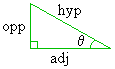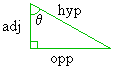Home    |    Teacher    |    Parents    |    Glossary    |    About UsEmail this page to a friendResources· Cool Tools · Formulas & Tables · References · Test Preparation · Study Tips · Wonders of MathSearchOverview ( Math | Algebra | Function |Overview)

Trig Functions: Overview

Under its simplest definition, a trigonometric (literally, a "triangle-measuring") function, is one of the many functions that relate one non-right angle of a right triangle to the ratio of the lengths of any two sides of the triangle (or vice versa).

Any trigonometric function (f), therefore, always satisfies either of the following equations:

f(q) = a / b   OR   f(a / b) = q,
where q is the measure of a certain angle in the triangle, and a and b are the lengths of two specific sides.

This means that

• If the former equation holds, we can choose any right triangle, then take the measurement of one of the non-right angles, and when we evaluate the trigonometric function at that angle, the result will be the ratio of the lengths of two of the triangle's sides.
• However, if the latter equation holds, we can choose any right triangle, then compute the ratio of the lengths of two specific sides, and when we evaluate the trigonometric function at any that ratio, the result will be measure of one of the triangles non-right angles. (These are called inverse trig functions since they do the inverse, or vice-versa, of the previous trig functions.)
This relationship between an angle and side ratios in a right triangle is one of the most important ideas in trigonometry. Furthermore, trigonometric functions work for any right triangle. Hence -- for a right triangle -- if we take the measurement of one of the triangles non-right angles, we can mathematically deduce the ratio of the lengths of any two of the triangle's sides by trig functions. And if we measure any side ratio, we can mathematically deduce the measure of one of the triangle's non-right angles by inverse trig functions. More importantly, if we know the measurement of one of the triangle's angles, and we then use a trigonometric function to determine the ratio of the lengths of two of the triangle's sides, and we happen to know the lengths of one of these sides in the ratio, we can then algebraically determine the length of the other one of these two sides. (i.e. if we determine that a / b = 2, and we know a = 6, then we deduce that b = 3.)

Since there are three sides and two non-right angles in a right triangle, the trigonometric functions will need a way of specifying which sides are related to which angle. (It is not-so-useful to know that the ratio of the lengths of two sides equals 2 if we do not know which of the three sides we are talking about. Likewise, if we determine that one of the angles is 40°, it would be nice to know of which angle this statement is true.

Under a certain convention, we label the sides as opposite, adjacent, and hypotenuse relative to our angle of interest q. full explanationAs mentioned previously, the first type of trigonometric function, which relates an angle to a side ratio, always satisfies the following equation:

f(q) = a / b.
Since given any angle q, there are three ways of choosing the numerator (a), and three ways of choosing the denominator (b), we can create the following nine trigonometric functions:

The three diagonal functions shown in red always equal one. They are degenerate and, therefore, are of no use to us. We therefore remove these degenerate functions and assign labels to the remaining six, usually written in the following order:

Furthermore, the functions are usually abbreviated: sine (sin), cosine (cos), tangent (tan) cosecant (csc), secant (sec), and cotangent (cot).

Do not be overwhelmed. By far, the two most important trig functions to remember are sine and cosine. All the other trig functions of the first kind can be derived from these two functions. For example, the functions on the right are merely the multiplicative inverse of the corresponding function on the left (that makes them much less useful). Furthermore, the sin(x) / COs(x) = (opp/hyp) / (adj/hyp) = opp / adj = tan(x). Therefore, the tangent function is the same as the quotient of the sine and cosine functions (the tangent function is still fairly handy).

 sine(q) = opp/hyp CSC(q) = 1/sin(q) COs(q) = adj/hyp sec(q) = 1/COs(q) tan(q) = sin(q)/COs(q) cot(q) = 1/tan(q)

Let's examine these functions further. You will notice that there are the sine, secant, and tangent functions, and there are corresponding "co"-functions. They get their odd names from various similar ideas in geometry. You may suggest that the cofunctions should be relabeled to be the multiplicative inverses of the corresponding sine, secant, and tangent functions. However, there is a method to this madness. A cofunction of a given trig function (f) is, by definition, the function obtained after the complement its parameter is taken. Since the complement of any angle q is 90° - q, the the fact that the following relations can be shown to hold:

sine(90° - q) = cosine(q)

secant(90° - q) = cosecant(q)
tangent(90° - q) = cotangent(q)
thus justifying the naming convention.

The trig functions evaluate differently depending on the units on q, such as degrees, radians, or grads. For example, sin(90°) = 1, while sin(90)=0.89399.... explanation

Just as we can define trigonometric functions of the form

f(q) = a / b
that take a non-right angle as its parameter and return the ratio of the lengths of two triangle sides, we can do the reverse: define trig functions of the form
f(a / b) = q
that take the ratio of the lengths of two sides as a parameter and returns the measurement of one of the non-right angles.

As before, the functions are usually abbreviated: arcsine (arcsin), arccosine (arccos), arctangent (arctan) arccosecant (arccsc), arcsecant (arcsec), and arccotangent (arccot). According to the standard notation for inverse functions (f-1), you will also often see these written as sin-1, cos-1, tan-1 csc-1, sec-1, and cot-1. Beware: There is another common notation that writes the square of the trig functions, such as (sin(x))2 as sin2(x). This can be confusing, for you then might then be lead to think that sin-1(x) = (sin(x))-1, which is not true. The negative one superscript here is a special notation that denotes inverse functions (not multiplicative inverses).